# The Remainder and Factor TheoremsPage 1

#### WATCH ALL SLIDES

Slide 1## The Remainder and Factor Theorems

Check for Understanding 3103.3.21 – Factor polynomials using a variety of methods including the factor theorem, synthetic division, long division, sums and differences of cubes, and grouping.

Slide 2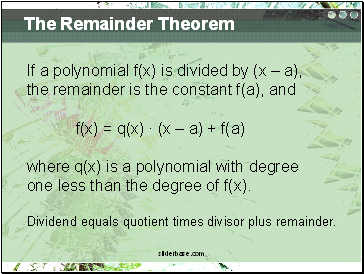## The Remainder Theorem

If a polynomial f(x) is divided by (x – a),

the remainder is the constant f(a), and

f(x) = q(x) ∙ (x – a) + f(a)

where q(x) is a polynomial with degree

one less than the degree of f(x).

Dividend equals quotient times divisor plus remainder.

Slide 3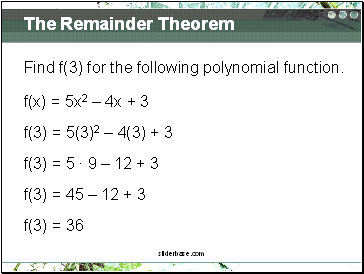The Remainder Theorem

Find f(3) for the following polynomial function.

f(x) = 5x2 – 4x + 3

f(3) = 5(3)2 – 4(3) + 3

f(3) = 5 ∙ 9 – 12 + 3

f(3) = 45 – 12 + 3

f(3) = 36

Slide 4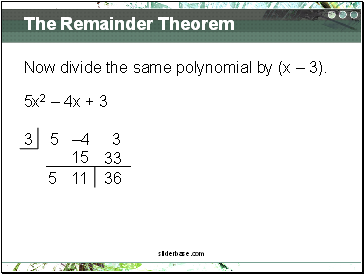The Remainder Theorem

Now divide the same polynomial by (x – 3).

5x2 – 4x + 3

3 5 –4 3

5

36

11

33

15

Slide 5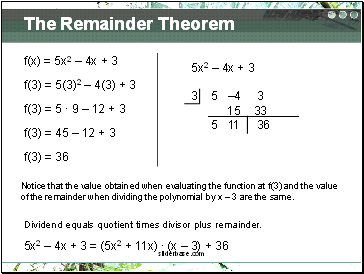The Remainder Theorem

5x2 – 4x + 3

3 5 –4 3

15 33

5 11 36

f(x) = 5x2 – 4x + 3

f(3) = 5(3)2 – 4(3) + 3

f(3) = 5 ∙ 9 – 12 + 3

f(3) = 45 – 12 + 3

f(3) = 36

Notice that the value obtained when evaluating the function at f(3) and the value

of the remainder when dividing the polynomial by x – 3 are the same.

Dividend equals quotient times divisor plus remainder.

5x2 – 4x + 3 = (5x2 + 11x) ∙ (x – 3) + 36

Slide 6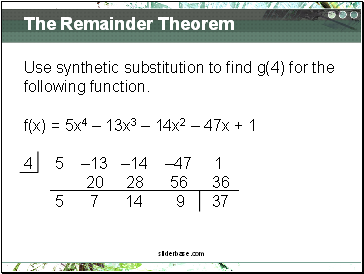The Remainder Theorem

Use synthetic substitution to find g(4) for the

following function.

f(x) = 5x4 – 13x3 – 14x2 – 47x + 1

4 5 –13 –14 –47 1

20 28 56 36

5 7 14 9 37

Slide 7The Remainder Theorem

Synthetic Substitution – using synthetic

division to evaluate a function

This is especially helpful for polynomials with

degree greater than 2.

Slide 8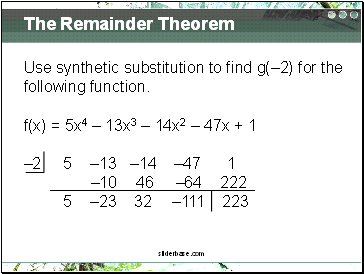The Remainder Theorem

Use synthetic substitution to find g(–2) for the

following function.

f(x) = 5x4 – 13x3 – 14x2 – 47x + 1

–2 5 –13 –14 –47 1

–10 46 –64 222

5 –23 32 –111 223

Slide 9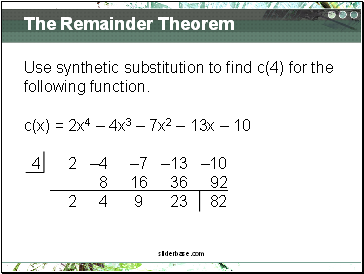The Remainder Theorem

Use synthetic substitution to find c(4) for the

following function.

c(x) = 2x4 – 4x3 – 7x2 – 13x – 10

Go to page:
1  2